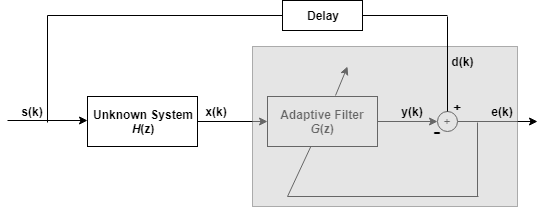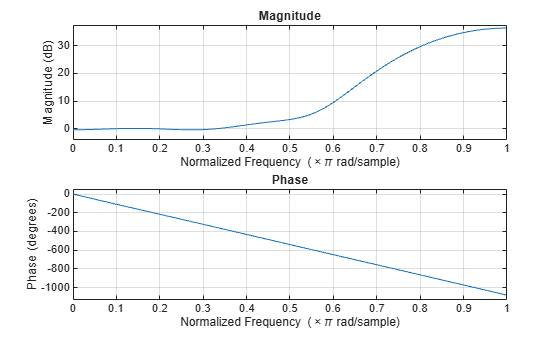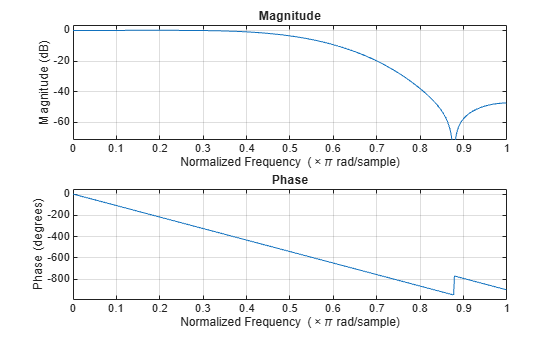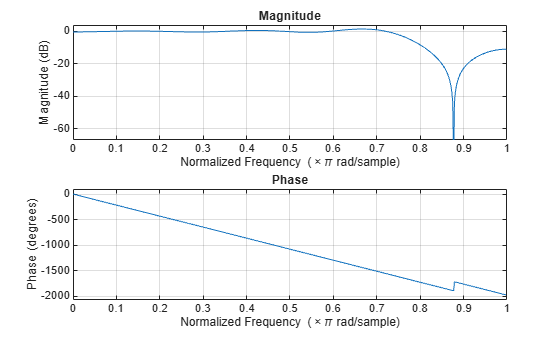Inverse System Identification Using RLS Algorithm

This example demonstrates the RLS adaptive algorithm using the inverse system identification model shown here.Cascading the adaptive filter with an unknown filter causes the adaptive filter to converge to a solution that is the inverse of the unknown system.

If the transfer function of the unknown system and the adaptive filter are H(z) and G(z), respectively, the error measured between the desired signal and the signal from the cascaded system reaches its minimum when G(z)$×$H(z) = 1. For this relation to be true, G(z) must equal 1/H(z), the inverse of the transfer function of the unknown system.

To demonstrate that this is true, create a signal s to input to the cascaded filter pair.

s = randn(3000,1);

In the cascaded filters case, the unknown filter results in a delay in the signal arriving at the summation point after both filters. To prevent the adaptive filter from trying to adapt to a signal it has not yet seen (equivalent to predicting the future), delay the desired signal by 12 samples, which is the order of the unknown system.

Generally, you do not know the order of the system you are trying to identify. In that case, delay the desired signal by number of samples equal to half the order of the adaptive filter. Delaying the input requires prepending 12 zero-value samples to the input s.

delay = zeros(12,1);
d = [delay; s(1:2988)]; % Concatenate the delay and the signal.

You have to keep the desired signal vector d the same length as x, so adjust the signal element count to allow for the delay samples.

Although not generally the case, for this example you know the order of the unknown filter, so add a delay equal to the order of the unknown filter.

For the unknown system, use a lowpass, 12th-order FIR filter.

filt = dsp.FIRFilter;
filt.Numerator = fir1(12,0.55,'low');

Filtering s provides the input data signal for the adaptive algorithm function.

x = filt(s);

To use the RLS algorithm, create a dsp.RLSFilter object and set its Length, ForgettingFactor, and InitialInverseCovariance properties.

p0 = 2 * eye(13);
lambda = 0.99;
rls = dsp.RLSFilter(13,'ForgettingFactor',lambda,...
'InitialInverseCovariance',p0);

This example seeks to develop an inverse solution, you need to be careful about which signal carries the data and which is the desired signal.

Earlier examples of adaptive filters use the filtered noise as the desired signal. In this case, the filtered noise (x) carries the unknown system's information. With Gaussian distribution and variance of 1, the unfiltered noise d is the desired signal. The code to run this adaptive filter is:

[y,e] = rls(x,d);

where y returns the filtered output and e contains the error signal as the filter adapts to find the inverse of the unknown system.

Obtain the estimated coefficients of the RLS filter.

b = rls.Coefficients;

View the frequency response of the adapted RLS filter (inverse system, G(z)) using freqz. The inverse system looks like a highpass filter with linear phase.

freqz(b,1)View the frequency response of the unknown system, H(z). The response is that of a lowpass filter with a cutoff frequency of 0.55.

freqz(filt.Numerator,1)The result of the cascade of the unknown system and the adapted filter is a compensated system with an extended cutoff frequency of 0.8.

overallCoeffs = conv(filt.Numerator,b);
freqz(overallCoeffs,1)Related Topics

 Hayes, Monson H., Statistical Digital Signal Processing and Modeling. Hoboken, NJ: John Wiley & Sons, 1996, pp.493–552.

 Haykin, Simon, Adaptive Filter Theory. Upper Saddle River, NJ: Prentice-Hall, Inc., 1996.

DSP System Toolbox DocumentationGet trial now